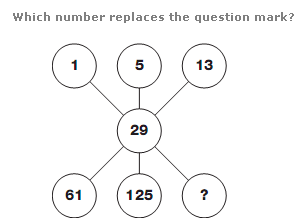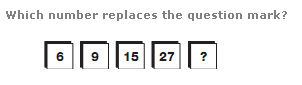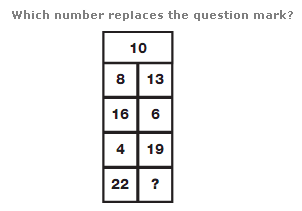# Puzzles - Number puzzles

Exercise : Number puzzles
19.:
253
Explanation
:
Starting at the top left, and moving through the diagram in a Z shape, double each number and add 3 to give the next number along.

20.:
51
Explanation
:
Moving to the right, double each number and subtract 3 to give the next number along.

21.